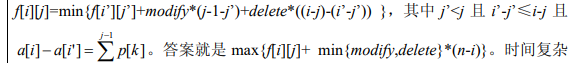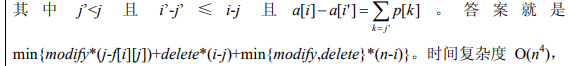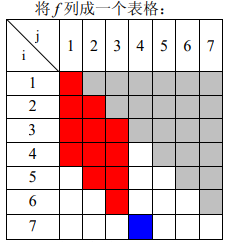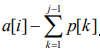# Title description

Description
Xiao Ming fell in love with stocks. After a period of observation and sorting, he discovered that if a stock has a certain shape of candlestick, then the stock will surely rise sharply in the near future. Xiao Ming wants to use this magic k-line to make a stock software. He expressed a k-line with an integer sequence a, and stipulated that if and only if a[i+1]-a[i]=p[i], this k-line is a magical k-line. But things are not always smooth sailing, Xiao Ming found that many candlesticks are not magical, but they can also rise sharply afterwards. But he found that these candlesticks are very close to the magical candlesticks. In order to further expand the use of the magic k-line, Xiao Ming defines the difference between the two k-lines b and a:
the cost of modifying an element in b to any value is cost1, and the cost of deleting an element in b is cost2 . The minimum cost sum of modifying the prefix of b to a is the degree of difference between b and a. The definition of the prefix here is a bit special, assuming that the length of b is m, and b is the prefix of a if and only if b[i+1]-b[i]=a[i+1]-a i .
The smaller the difference between a k-line and the magical k-line, the higher the probability of a big increase.
Although Xiaoming himself can quickly calculate the difference between a certain k-line and a magical k-line, if you want to make software, you have to write a program to calculate it. Can you help him?

Input the
first line of three positive integers n, cost1, cost2. n represents the length of the given k-line a, and the meanings of cost1 and cost2 are as in the title.
The n-1 integers in the second line represent p to p[n-1] in turn, with the same meaning as the title.
The n integers in the third row represent n elements in the given k-line a in turn.

Output
a number, the degree of difference between a and the magical k-line.

Sample Input
8 1 2
1 2 3 4 5 6 7
0 1 999 6 10 -999 15 21

Sample Output
3

Data Constraint

Hint
[Sample explanation] Change
999 to 3, delete -999, and get the sequence 0 1 3 6 10 15 21. There is no less costly solution.
[Data range]
For 30% data: n<=100
For 60% data: n<=500
For 100% data: n<=1500
cost1, cost2<=1000000
The absolute value of each element in p is < =1000
The absolute value of each element in a is <=1000000

First consider a naive dp:
let f[i][j] denote that the i-th element in a is retained, and the minimum cost of the new sequence length j is obtained.
Transfer:Consider changing the meaning of f[i][j], set f[i][j] indicates that the i-th element in a is retained, and the initial value of the maximum retained number of the new sequence length j is obtained: f[i][j]=1, because it can be modified + deleted to make a[ i] becomes the end of the length 1~a[i] and the
transition is so long. Itcan be found that if the beginnings of the two sequences are different, then the transitions of the two sequences will not affect each other
, that is, thedifferences are
classified according to this category, and between the same category There is no limit to transfer,
so it seems to be O(n 3 )

Maintain the maximum value of each column in the same category, press (ij) from small to large, j from small to large, monotonous maintenance + line segment tree
time: O(n 2 log n)

# code

``````#include <algorithm>
#include <iostream>
#include <cstdlib>
#include <cstring>
#include <cstdio>
#define fo(a,b,c) for (a=b; a<=c; a++)
#define fd(a,b,c) for (a=b; a>=c; a--)
#define min(a,b) (a<b?a:b)
#define max(a,b) (a>b?a:b)
using namespace std;

struct type{

int x,y,s,first;
} A;
int a;
int p;
int tr;
int n,mod,del,i,j,k,l,ans,tot,len,s;

bool cmp(type a,type b)
{

return a.first<b.first || a.first==b.first && a.s<b.s || a.first==b.first && a.s==b.s && a.y<b.y;
}

void clear(int t)
{

tr[t]=0;
tr[t]=0;
tr[t]=-2133333333;
}

void New(int t,int x)
{

if (!tr[t][x])
{

tr[t][x]=++len;
clear(len);
}
}

void change(int t,int l,int r,int x,int s)
{

int mid=(l+r)/2;

tr[t]=max(tr[t],s);

if (l==r)
return;

if (x<=mid)
{

New(t,0);
change(tr[t],l,mid,x,s);
}
else
{

New(t,1);
change(tr[t],mid+1,r,x,s);
}
}

int find(int t,int l,int r,int x,int y)
{

int mid=(l+r)/2,ans=-2133333333,s;

if (x<=l && r<=y)
return tr[t];

if (x<=mid)
{

if (tr[t])
{

s=find(tr[t],l,mid,x,y);
ans=max(ans,s);
}
}
if (mid<y)
{

if (tr[t])
{

s=find(tr[t],mid+1,r,x,y);
ans=max(ans,s);
}
}

return ans;
}

int main()
{

// 	freopen("a.in","r",stdin);
// 	freopen("b.out","w",stdout);
//	freopen("kei2.in","r",stdin);
//	freopen("S8_7_3.in","r",stdin);

scanf("%d%d%d",&n,&mod,&del);
fo(i,1,n-1)
{

scanf("%d",&p[i]);
p[i]+=p[i-1];
}
fo(i,1,n)
scanf("%d",&a[i]);

fo(i,1,n)
{

fo(j,1,i)
{

++tot;
A[tot].x=i;
A[tot].y=j;
A[tot].s=i-j;
A[tot].first=a[i]-p[j-1];
}
}

sort(A+1,A+tot+1,cmp);

ans=min(del,mod)*n;

len=1;
clear(1);

fo(l,1,tot)
{

if (A[l].y>1)
s=find(1,1,n,1,A[l].y-1);
else
s=-2133333333;
s=max(s+1,1);

ans=min(ans,(A[l].x-A[l].y)*del+(A[l].y-s)*mod+min(del,mod)*(n-A[l].x));

change(1,1,n,A[l].y,s);

if (l<tot && A[l].first!=A[l+1].first)
{

len=1;
clear(1);
}
}

printf("%d\n",ans);
}
``````

### Guess you like

Origin blog.csdn.net/gmh77/article/details/99549599
Recommended
Ranking
Daily